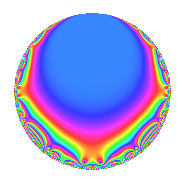# Properties

 Label 6003.2.a.dLevel 6003 Weight 2 Character orbit 6003.a Self dual Yes Analytic conductor 47.934 Analytic rank 1 Dimension 2 CM No Inner twists 1

# Related objects

## Newspace parameters

 Level: $$N$$ = $$6003 = 3^{2} \cdot 23 \cdot 29$$ Weight: $$k$$ = $$2$$ Character orbit: $$[\chi]$$ = 6003.a (trivial)

## Newform invariants

 Self dual: Yes Analytic conductor: $$47.9341963334$$ Analytic rank: $$1$$ Dimension: $$2$$ Coefficient field: $$\Q(\sqrt{17})$$ Coefficient ring: $$\Z[a_1, \ldots, a_{5}]$$ Coefficient ring index: $$1$$ Fricke sign: $$1$$ Sato-Tate group: $\mathrm{SU}(2)$

## $q$-expansion

Coefficients of the $$q$$-expansion are expressed in terms of $$\beta = \frac{1}{2}(1 + \sqrt{17})$$. We also show the integral $$q$$-expansion of the trace form.

 $$f(q)$$ $$=$$ $$q - q^{2} - q^{4} + ( 1 + \beta ) q^{5} + 3 q^{8} +O(q^{10})$$ $$q - q^{2} - q^{4} + ( 1 + \beta ) q^{5} + 3 q^{8} + ( -1 - \beta ) q^{10} + ( 1 + \beta ) q^{11} + ( -1 - \beta ) q^{13} - q^{16} -2 \beta q^{19} + ( -1 - \beta ) q^{20} + ( -1 - \beta ) q^{22} - q^{23} + 3 \beta q^{25} + ( 1 + \beta ) q^{26} + q^{29} + ( -5 + \beta ) q^{31} -5 q^{32} + ( 5 - \beta ) q^{37} + 2 \beta q^{38} + ( 3 + 3 \beta ) q^{40} + ( -5 - \beta ) q^{41} + ( 6 - 4 \beta ) q^{43} + ( -1 - \beta ) q^{44} + q^{46} + ( -2 - 2 \beta ) q^{47} -7 q^{49} -3 \beta q^{50} + ( 1 + \beta ) q^{52} + ( -6 + 4 \beta ) q^{53} + ( 5 + 3 \beta ) q^{55} - q^{58} + ( -7 - \beta ) q^{59} + ( 1 - 5 \beta ) q^{61} + ( 5 - \beta ) q^{62} + 7 q^{64} + ( -5 - 3 \beta ) q^{65} + ( 9 - \beta ) q^{67} + ( -13 + \beta ) q^{71} -2 \beta q^{73} + ( -5 + \beta ) q^{74} + 2 \beta q^{76} -2 q^{79} + ( -1 - \beta ) q^{80} + ( 5 + \beta ) q^{82} + ( 2 + 2 \beta ) q^{83} + ( -6 + 4 \beta ) q^{86} + ( 3 + 3 \beta ) q^{88} + ( -2 - 6 \beta ) q^{89} + q^{92} + ( 2 + 2 \beta ) q^{94} + ( -8 - 4 \beta ) q^{95} + ( 2 - 6 \beta ) q^{97} + 7 q^{98} +O(q^{100})$$ $$\operatorname{Tr}(f)(q)$$ $$=$$ $$2q - 2q^{2} - 2q^{4} + 3q^{5} + 6q^{8} + O(q^{10})$$ $$2q - 2q^{2} - 2q^{4} + 3q^{5} + 6q^{8} - 3q^{10} + 3q^{11} - 3q^{13} - 2q^{16} - 2q^{19} - 3q^{20} - 3q^{22} - 2q^{23} + 3q^{25} + 3q^{26} + 2q^{29} - 9q^{31} - 10q^{32} + 9q^{37} + 2q^{38} + 9q^{40} - 11q^{41} + 8q^{43} - 3q^{44} + 2q^{46} - 6q^{47} - 14q^{49} - 3q^{50} + 3q^{52} - 8q^{53} + 13q^{55} - 2q^{58} - 15q^{59} - 3q^{61} + 9q^{62} + 14q^{64} - 13q^{65} + 17q^{67} - 25q^{71} - 2q^{73} - 9q^{74} + 2q^{76} - 4q^{79} - 3q^{80} + 11q^{82} + 6q^{83} - 8q^{86} + 9q^{88} - 10q^{89} + 2q^{92} + 6q^{94} - 20q^{95} - 2q^{97} + 14q^{98} + O(q^{100})$$

## Embeddings

For each embedding $$\iota_m$$ of the coefficient field, the values $$\iota_m(a_n)$$ are shown below.

For more information on an embedded modular form you can click on its label.

Label $$\iota_m(\nu)$$ $$a_{2}$$ $$a_{3}$$ $$a_{4}$$ $$a_{5}$$ $$a_{6}$$ $$a_{7}$$ $$a_{8}$$ $$a_{9}$$ $$a_{10}$$
1.1
 −1.56155 2.56155
−1.00000 0 −1.00000 −0.561553 0 0 3.00000 0 0.561553
1.2 −1.00000 0 −1.00000 3.56155 0 0 3.00000 0 −3.56155
 $$n$$: e.g. 2-40 or 990-1000 Significant digits: Format: Complex embeddings Normalized embeddings Satake parameters Satake angles

## Inner twists

This newform does not admit any (nontrivial) inner twists.

## Atkin-Lehner signs

$$p$$ Sign
$$3$$ $$-1$$
$$23$$ $$1$$
$$29$$ $$-1$$

## Hecke kernels

This newform can be constructed as the intersection of the kernels of the following linear operators acting on $$S_{2}^{\mathrm{new}}(\Gamma_0(6003))$$:

 $$T_{2} + 1$$ $$T_{5}^{2} - 3 T_{5} - 2$$